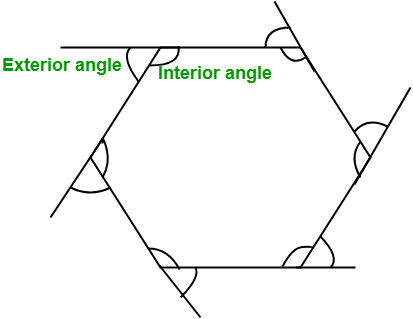# Program to find the Interior and Exterior Angle of a Regular Polygon

Given the number of sides n of a regular polygon. The task is to find out the Interior angle and Exterior angle of the polygon.

Examples:

Input : n = 6
Output : Interior angle: 120
Exterior angle: 60

Input : n = 10
Output: Interior angle: 144
Exterior angle: 36


## Recommended: Please try your approach on {IDE} first, before moving on to the solution.

Interior angle: The angle between two adjacent sides inside the polygon is known as the Interior angle.
Formula to find the Interior angle:

Interior Angle =Exterior angle: The angle formed by any side of a polygon and the extension of its adjacent side is known as Exterior angle.

Exterior angle =Program to find interior and exterior angles of a Regular Polygon:

## C++

 // CPP program to find the interior and   // exterior angle of a given polygon  #include  using namespace std;     // function to find the interior and  // exterior angle  void findAngle(int n)  {      int interiorAngle, exteriorAngle;         // formula to find the interior angle      interiorAngle = (n - 2) * 180 / n;             // formula to find the exterior angle      exteriorAngle = 360 / n;         // Displaying the output      cout << "Interior angle: " << interiorAngle << endl;         cout << "Exterior angle: " << exteriorAngle;  }     // Driver code  int main()  {      int n = 10;             // Function calling      findAngle(n);      return 0;  }

## Java

 // Java program to find the interior and   // exterior angle of a given polygon  import java.io.*;     class GFG {             // function to find the interior and      // exterior angle      static void findAngle(int n)      {          int interiorAngle, exteriorAngle;                 // formula to find the interior angle          interiorAngle = (n - 2) * 180 / n;                     // formula to find the exterior angle          exteriorAngle = 360 / n;                 // Displaying the output          System.out.println("Interior angle: " + interiorAngle);                 System.out.println("Exterior angle: " + exteriorAngle);      }             // Driver code      public static void main (String[] args)      {          int n = 10;                 // Function calling          findAngle(n);      }  }

## Python3

 # Python3 program to find   # the interior and exterior  # angle of a given polygon     # function to find   # the interior and  # exterior angle  def findAngle(n):         # formula to find the      # interior angle      interiorAngle = int((n - 2) * 180 / n)             # formula to find       # the exterior angle      exteriorAngle = int(360 / n)         # Displaying the output      print("Interior angle: " ,              interiorAngle )         print("Exterior angle: " ,              exteriorAngle )     # Driver code  n = 10        # Function calling  findAngle(n)     # This code is contributed   # by Smitha

## C#

 // C# program to find the   // interior and exterior  // angle of a given polygon  using System;     class GFG   {             // function to find      // the interior and      // exterior angle      static void findAngle(int n)      {          int interiorAngle,               exteriorAngle;                 // formula to find           // the interior angle          interiorAngle = (n - 2) * 180 / n;                     // formula to find           // the exterior angle          exteriorAngle = 360 / n;                 // Displaying the output          Console.Write("Interior angle: " +                      interiorAngle + "\n");                 Console.Write("Exterior angle: " +                              exteriorAngle);      }             // Driver code      public static void Main ()      {          int n = 10;                 // Function calling          findAngle(n);      }  }     // This code is contributed  // by Smitha

## PHP

 

Output:

Interior angle: 144
Exterior angle: 36


Attention reader! Don’t stop learning now. Get hold of all the important DSA concepts with the DSA Self Paced Course at a student-friendly price and become industry ready.

My Personal Notes arrow_drop_upCheck out this Author's contributed articles.

If you like GeeksforGeeks and would like to contribute, you can also write an article using contribute.geeksforgeeks.org or mail your article to contribute@geeksforgeeks.org. See your article appearing on the GeeksforGeeks main page and help other Geeks.

Please Improve this article if you find anything incorrect by clicking on the "Improve Article" button below.

Article Tags :
Practice Tags :

Be the First to upvote.

Please write to us at contribute@geeksforgeeks.org to report any issue with the above content.